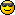Discussion about math, puzzles, games and fun.   Useful symbols: ÷ × ½ √ ∞ ≠ ≤ ≥ ≈ ⇒ ± ∈ Δ θ ∴ ∑ ∫  π  -¹ ² ³ °

You are not logged in.

#1 2005-06-03 01:26:16

cvd
Member
Registered: 2005-06-03
Posts: 1

Work these equations out!

Work these 'Equations of Straight Lines' out!

First of all, the rule is:
*USA* y = mx+b
*UK* y = mx+c

Work these out:

(a) (2,-1),(4,2)
(b) (-3,1),(-1,-1)
(c) (2,1),(6,1)
(d) (-1/2,1),(2,1/2)
(e) (0,4),(-2,1)

Have fun working them out~*

Offline

#2 2005-06-03 11:20:05

MathsIsFunRegistered: 2005-01-21
Posts: 7,694

Re: Work these equations out!

In your first example you have (2,-1),(4,2)

That really means you have two points that you KNOW. The first point is (2,-1), and the second is (4,2).

When we write down a point as (2,-1), the first number is assumed to be the "along" or horizontal value (which we usually call x), and the second number is the up-down value (called y).

So we could restate the problem in simple english as:

What is the line that passes through the point 2 spaces along and 1 space down (-1 is down) and also through the point 4 spaces along and 2 spaces up.

OK, you probably knew all that, so on with the meaty stuff.

First you work out the slope, which is how fast the line rises.

Well, we know the two points (2,-1),(4,2), so we can see that the line moves along from 2 to 4 while moving from 1 space down to 2 up.

So it moves along (4-2)=2 spaces while moving up (2-(-1))=3 spaces. So the slope is 3/2.

(Just think, if you were walking uphill, and you went up 1 meter for every 10 meters forward, that is a light slope upwards, and is a 1/10 slope, but if you had to go up 3m for every 2m along that would be a steep slope of 3/2)

The formula we just used is: m (the slope) = (y2 - y1) / (x2 - x1)

In other words m = (2-(-1)) / (4-2) = 3/2.

Next you work out the "intercept", which is where the line crosses through x=0 (which is the y axis if you draw a proper graph)

Well, we know that the slope is 3/2, so we can just start at any point and figure where the line would be when x=0

So, let's start at the first point (2,-1) (2 steps along, one down)

And we know that the line moves 3 steps up for every 2 steps along. But we need to move 2 steps BACK to get to zero, so in the same time the line must move 3 steps DOWN.

So, the y-value (the up-down value) started at "one down" and moved a further 3 steps down, so it must be at 4 steps down when x is at 0.

The formula we just used is: b (the intercept) = y1 - (m * x1) in other words, start at y1 and travel backwards along the slope x1 steps, because we want x1 to be zero.

m (the slope) = 3/2
b (the intercept) = -4

This can be written even more neatly as y = (3/2)x - 4

Proof: at 2, y = 1.5*2-4 = 3-4 = -1, at 4, y = 1.5*4-4 = 6-4 = 2

Your other solutions can be done the same way, but because we now have the formulas to work them out, we can just trust to mathematics that they will work.

(b) (-3,1),(-1,-1)

m (the slope) = (y2 - y1) / (x2 - x1) = (-1-1) / (-1-(-3)) = (-2)/(-1+3) = -2/2 = -1
b (the intercept) = y1 - (m * x1) = 1 - (-1)*-3 = 1 - 3 = -2

y = -1x-2

(c) (2,1),(6,1)

m (the slope) = (y2 - y1) / (x2 - x1) = (1-1) / (6-2) = 0/4 = 0
b (the intercept) = y1 - (m * x1) = 1 - 0*2 = 1

y = 0x + 1 = 1  (ie the equation of the line is just "y=1", no matter what value x is, y will always equal 1)

Hey, you can do the rest for practice !

"The physicists defer only to mathematicians, and the mathematicians defer only to God ..."  - Leon M. Lederman

Offline

#3 2005-06-22 09:00:44

Mr T
MemberRegistered: 2005-03-30
Posts: 1,012

Re: Work these equations out!

yay for the U.KI come back stronger than a powered-up Pac-ManI bought a large popcorn @ the cinema the other day, it was pretty big...some might even say it was "largeFatboy Slim is a LegendOffline

#4 2019-05-07 23:20:11

Alg Num Theory
MemberRegistered: 2017-11-24
Posts: 693
Website

Re: Work these equations out!

The most general equation of the straight line passing through the points (a₁,b₁) and  (a₂,b₂) (where either a₁ ≠ a₂ or b₁ ≠ b₂ (or both)) is:

[list=*]
[*]

.[/*]
[/list]

If a₁ = a₂, you get the vertical line x = a₁. If a₁ ≠ a₂, you get a line of the form y = mx +c.

Me, or the ugly man, whatever (3,3,6)

Offline